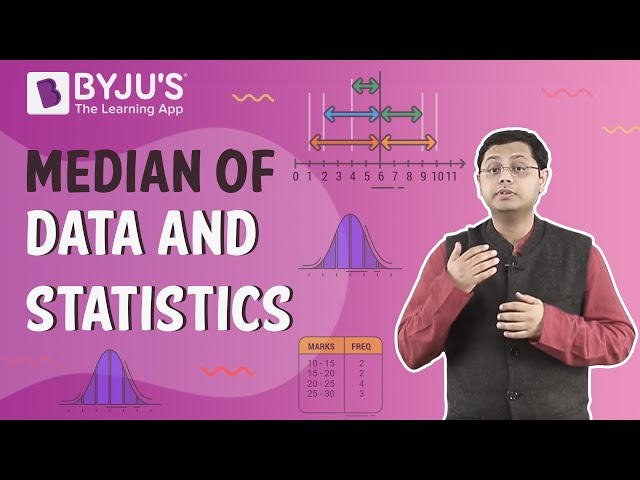# Difference Between Mean and Median

The difference between mean and median is explained in detail here. In statistics, mean is the average of a set of data and the median is the middle value of the arranged set of data. Both values have their own importance and play a distinct role in data collection and organisation. Let us see what are other differences between them with the help of definitions, table and example.

## Definition of Mean and Median

Mean: Mathematically. the mean can simply be defined as the list of numbers that are used to describe a central tendency. The method to calculate mean is simple enough, the variables need to be added and divided by the number of items in the overall sample. Assume a data value, say x1, x2, … , xn

So, the formula for calculating the mean is given as:

Mean =  (x1+ x2+ … + xn)/n

Here “n” is the total number of sample items.

Median: Median, on the other hand, can simply be defined as the number that is found in the middle of the set. Median is an essential quantity that can be used for separating the available sample into two; the higher half sample, as well as the lower half sample, can be procured in this method.

To proceed with the process of finding the median of the given data, first, arrange the given set of numbers on the ascending order, and then determine the middle value from the centre of the distribution. This condition can be suitable if we have an odd number of observations. But, in case of even number of observation, there is no single median value. So, in this case, add the two numbers in the middle and then divide it by 2. The obtained value is taken as a median value.

## What is the Difference between Mean and Median?

Here, the major difference between mean and median is listed below. Go through the following differences.

Difference between Mean and Median
Mean Median
The average arithmetic of a given set of numbers is called Mean. The method of separating the higher sample with the lower value, usually from a probability distribution is termed as the median
The application for the mean is for normal distributions The primary application for the median is skewed distributions.
There are a lot of external factors that limit the use of Mean. It is much more robust and reliable for measuring the data for uneven data.
Mean  can found by calculated by adding all the values and dividing the total by the number of values. Median can be found by listing all the numbers available in the set in arranging the order and then finding the number in the centre of the distribution.
Mean is considered as an arithmetic average. Median is considered as a positional average.
It is highly sensitive to outlier data It is not much sensitive to the outlier data.
It defines the central value of the data set. It defines the centre of gravity of the midpoint of the data set.

Thus, these are the major differences between Mean and Median. It is essential to know the major differences between the two.

### Solved Example

Question: Find the mean and median for the following data:

3, 5, 4, 1, 8, 6, 9

Solution:

Given Data: 3, 5, 4, 1, 8, 6, 9

No. of. data = 7

The mean for the following data is given as:

Mean =  (3 +5 + 4 + 1 + 8 + 6 + 9)/7

Mean = 36/7 = 5. 14

Therefore, the mean of the following data is 5. 14

To find median:

Step 1: Arrange the given set of data in ascending order

So, it becomes: 1, 3, 4, 5, 6, 8, 9

Step 2: Since the number of data is odd, take the middle value

So, the middle value of the given data is 5.

Thus, the median value is 5.

## Video Lesson on Median of Data## Frequently Asked Questions on Mean and Median

Q1

### What is the difference between mean and median?

Mean is the average value of set of given data and median is the middle value when the data set is arranged in an order either ascending or descending.
Q2

### How to find mean and median?

Mean is equal to ratio of sum of all the values in a data set and total number of values.
To find median we need to arrange the given values in ascending order or in descending order, then find the middle value.
Q3

### What is the formula for mean, median and mode?

Mean = Sum of Observation/Total number of observation
After arranging the values in an order and if the total number of observation is odd then the formula for median is:
Median = {(n+1)/2}th term
If the total number of observation is even then the formula to calculate the median is:
Median = [(n/2)th term + {(n/2)+1}th]/2
Mode = Value which is repeated most of the times in an observation.
Q4

### What is the difference between median and mode?

The mode states the frequency of an observation or the number of times a value is repeated in a data set.
Median is the middle value of the given data or observations when arranged in an order.
Test your knowledge on Difference Between Mean And Median